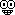NEW PROBLEMS...NEW PROBLEMS...

1979 BC 7

Let f be a function with domain the set of all real numbers and having the following properties:
(i) f(x + y) = f(x)f(y) for all real numbers x and y.
(ii) limh→0 [f(h) - 1]/h = k, where k is a nonzero real number.

(a) Use these properties and a definition of the derivative to show that f'(x) exists for all real numbers x.

(b) Let f(n) denote the nth derivative of f. Write an expression for f(n)(x) in terms of f(x).

(c) Given that f(1) = 2, use the Mean Value Theorem to show that there exists a number c such that 0< c< 3 and f'(c) = 7/3.

1981 BC 6
(a) A solid is constructed so that it has a circular base of radius r centimeters and every plane section perpendicular to a certain diameter of the base is a square, with a side of the square being a chord ofthe circle. Find the volume of the solid.

(b) If the solid described in part (a) expands so that the radius of the base increases at a constant rate of 1/2 centimeters per minute, how fast is the volume changing when the radius is 4 centimeters?Supreme Math GOD

Posts : 22
Join date : 2009-03-19
Age : 37Re: NEW PROBLEMS...

ahh i dont have internet at home anymore!!

so i am gonna copy all these problems down and then work on them and show you at the next study session.

BTW, WHEN IS THE NEXT STUDY SESSION? PLEASE TEXT ME SOMEONE LOLOLshakalohana

Posts : 17
Join date : 2010-06-04Permissions in this forum:
You cannot reply to topics in this forum